# Python: Logistic Regression

This lesson will focus more on performing a Logistic Regression in Python. If you are unfamiliar with Logistic Regression, check out my earlier lesson: Logistic Regression with Gretl

## Import the Data

You should be good at this by now, use Pandas .read_excel().

df.head() gives us a the first 5 rows.

What we have here is a list of students applying to a school. They have a Score that runs from 0 -1600,  ExtraCir (extracurricular activity) 0 = no 1 = yes, and finally Accepted 0 = no 1 = yes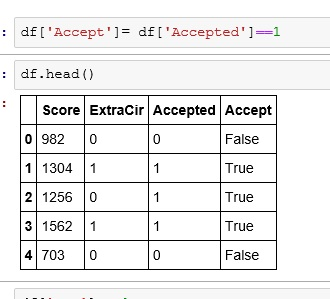## Create Boolean Result

We are going to create a True/False column for our dataframe.

What I did was:

• df[‘Accept’]   — create a new column named Accept
• df[‘Accepted’]==1  — if my Accepted column is 1 then True, else False## What are we modeling?

The goal of our model is going to be to predict and output – whether or not someone gets Accepted based on some input – Score, ExtraCir.

So we feed our model 2 input (independent)  variables and 1 result (dependent) variable. The model then gives us coefficients. We place these coefficients(c,c1,c2) in the following formula.

y = c + c1*Score + c2*ExtraCir

Note the first c in our equation is by itself. If you think back to the basic linear equation (y= mx +b), the first c is b or the y intercept. The Python package we are going to be using to find our coefficients requires us to have a place holder for our y intercept. So, let’s do that real quick.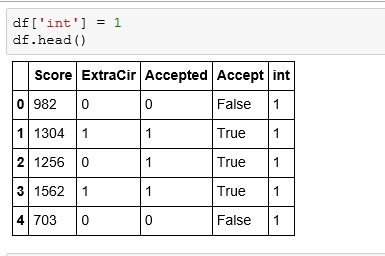## Let’s build our model

Let’s import statsmodels.api

From statsmodels we will use the Logit function. First giving it the dependent variable (result) and then our independent variables.

After we perform the Logit, we will perform a fit()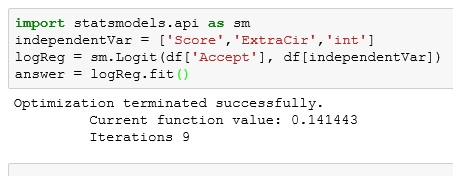The summary() function gives us a nice chart of our resultsIf you are a stats person, you can appreciate this. But for what we need, let us focus on our coef.remember our formula from above: y = c + c1*Score + c2*ExtraCir

Let’s build a function that solves for it.

Now let us see how a student with a Score of 1125 and a ExCir of 1 would fair.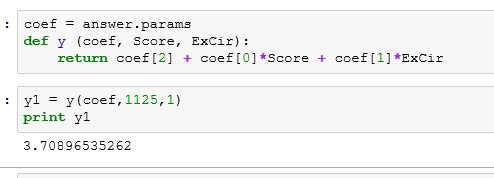okayyyyyy. So does 3.7089 mean they got in?????

Let’s take a quick second to think about the term logistic. What does it bring to mind?

Logarithms!!!

Okay, but our results equation was linear — y = c+ c1*Score + c2*ExCir

So what do we do.

So we need to remember y is a function of probability.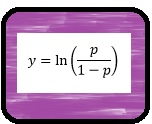So to convert y our into a probability, we use the following equationSo let’s import numpy so we can make use of e (exp() in Python)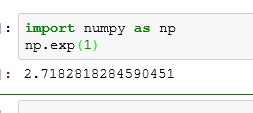Run our results through the equation. We get .97. So we are predicting a 97% chance of acceptance.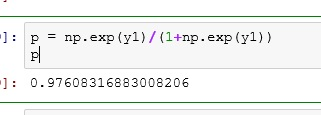Now notice what happens if I drop the test score down to 75. We end up with only a 45% chance of acceptance.If you enjoyed this lesson, click LIKE below, or even better, leave me a COMMENT.

## 4 thoughts on “Python: Logistic Regression”

1.agalea91

Nice post Ben. The one thing that seems to drop from the sky is the equation y=log (p/(1-p)), where does this come from?

1.Ben Larson

Thanks for the comment.

I will go back and edit the page to clear this up. But for now, here is a brief explanation. p = probability. p/(1-p) is a well known equation called the odds ratio. Taking the natural log (ln) of the odds ratio gives us the logit function. The logit function (which is actually the inverse of logistic function when you graph it) has a special property in regression. It can be used to link our linear function: y = mx + b with our probability (p).

1.agalea91

Thank you!

2.Minerva

I believe this website has very good written subject material
articles. http://renato-usatyi-novosti.com